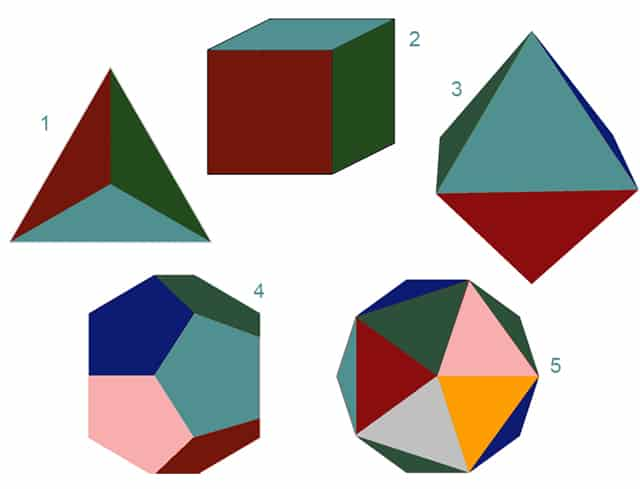Platonic Solid Transformation by Common NumbersPlatonic Solid Transformation by Common Numbers. There are 5 regular polyhedrons. They have a special property called duality. In geometry, any polyhedron is associated with a second dual figure. The vertices of one correspond to the faces of the other. The edges between pairs of vertices of one correspond to the edges between pairs of faces of the other. There are only 5 dual regularly polyhedrons. A 6th cannot be constructed.Platonic Solid Transformation- Here it is:

The cube and octahedron are dual. Note the 6 corners of the octahedron. All 6 corners can be inscribed mid-face in the cube. The opposite construction is also true. The cube has 8 corners. It can be inscribed in the 8 faces of the octahedron. Note the equivalency on the featured picture of the cube and octahedron. Eight faces of the octahedron become the 8 corners of the cube. Six faces of the cube become the six corners of the octahedron. Here is where we challenge the mind of modern man. With the regular polyhedrons, numbers carry the essence of geometry. The cube and octahedron, they seemingly transform corners to edges as if by magic. In the process an octahedron becomes a cube. Or, in reverse, a cube becomes an octahedron. In the exact same way, the icosahedron is dual to the dodecahedron. The 12 faces of the dodecahedron become the 12 corners of the cube. The 20 faces of the icosahedron become the 20 corners of the dodecahedron. In these  two pairs of examples, numbers become the vehicles of geometrical exchange. Thus, numbers have an even higher reality than geometry. Pythagoras was correct.

Sir William SmithDictionary of Greek and Roman Biography and Mythology (1870, p. 620).since other things seemed in their entire nature to be formed in the likeness of numbers, and in all nature numbers are the first.  They supposed the elements of numbers to be the elements of all things“.  Pythagoreans recognized in numbers the inherent basis of things. From the common conviction that it was in numbers and their relations they were to find principles of knowledge. They regarded the principles of numbers as the absolute principles of things.

The tetrahedron is the glaring exception. This Platonic solid transformation is self-dual. It is dual to itself. When faces and corners reverse, another tetrahedron is formed. Only, it is up-side-down. Below is a picture of how this self dual figure outlines the boundaries of the cube. The tetrahedron has 4 corners, 6 edges and 4 faces. On purpose, the tetrahedron topological features are coded in the Hebraic spelling of the name, David. Hebrew: דָּוִד

With David, dalet is the symbol for 4. Vav is the symbol for 6. And we have a 2nd dalet for another 4. In Hebrew the letters doubled as numbers. So it also alludes to the 1st and only unchageable polyhedron. It does not transform. It is only reversed.

Finally, note the total number degrees of the 5 solids in the featured picture. It is 14,400. That numbers the feet in a megalithic mile. This is not a coincidence. Ancient wisdom was at least in part based on a thorough understanding of these principles. Is modern man really all that advanced?A tetrahedron is the only regular polyhedron that is dual to itself.#1 = tetrahedron. #2 = cube. #3 = octahedron. #4 = dodecaedron. # 5 = icosahedron.